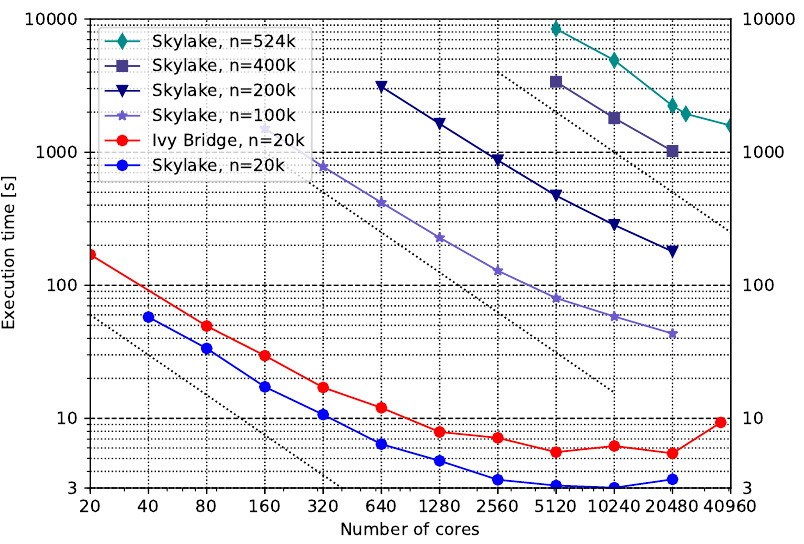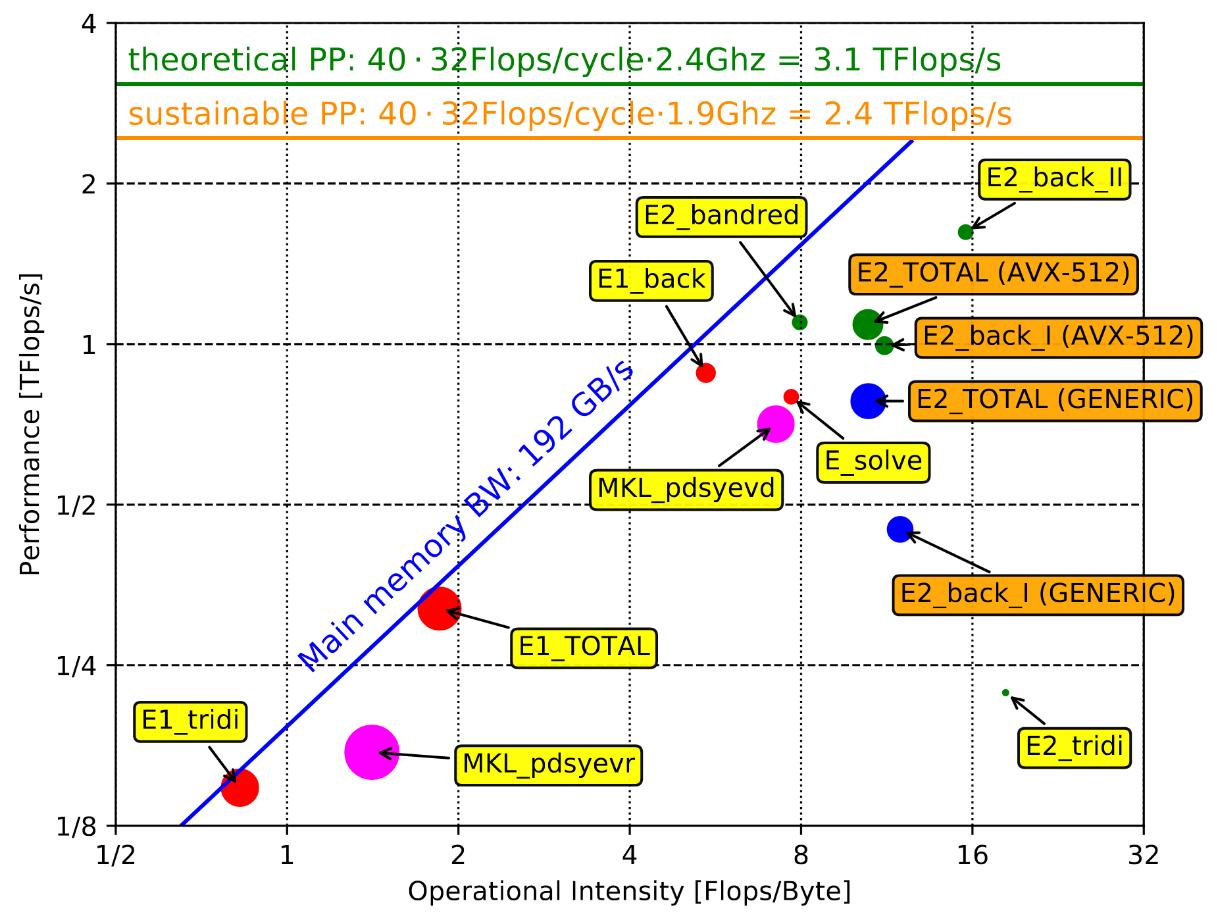# Intel Skylake¶

On the MPCDF HPC system Cobra (Intel Xeon Gold 6148, 20 cores @ 2.4 GHz, 100 Gb/s OmniPath interconnect) the following scaling results have been achieved and published. The results have been obained with the ELPA version 2017.11.001.

## Strong scaling¶Fig 1: Scaling of the 2-stage solver of the ELPA library for different real, double-precision matrix sizes (100% of eigenvectors sought). The red line shows the scaling on a older Intel Ivy Bridge (2.6 Ghz, 2x8 cores per node, FDR14 Infiniband) system, to demonstrate the performance improvements of the ELPA-library.

## Roofline model¶Fig 2: A roofline model for the ELPA 1stage (red), ELPA 2stage (blue and green), and the Intel MKL implementation of the routines “pdsyevr” and “pdsyevd” (purple). The size of the colored circles is proportional to the needed runtime. For ELPA 1stage data points are shown for the three algorithmic steps “tridiagonalization” (denoted E1_tridi), “solve” (denoted E_solve), and “back-transformaton” (denoted E1_back”) and the total runtime (denoted E1_TOTAL). For ELPA 2stage data points are shown for the five algorithmic steps “transformation to band-matrix” (denoted E2_bandred), “transformation to tridiagonal form” (denoted E2_tridi), “solve” (denoted E_solve), “backtransformation to banded form” (denoted E2_back_I), “backtransformation to full form” (denoted E2_back_II) and the total runtime (denoted E2_TOTAL). For the first back transformation and the total runtime, the performance for a generic implementation (denoted GENERIC) and for a AVX-512 optimized implementation (denoted AVX-512) are shown.